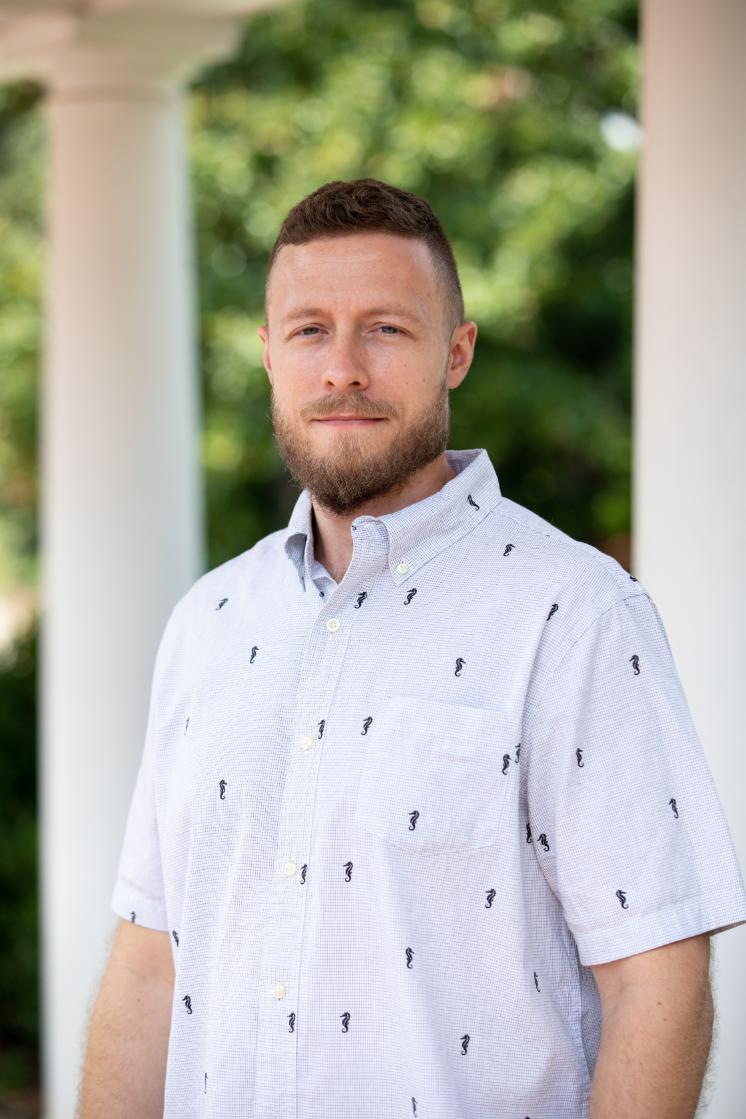# Steven HoehnerAsst Prof, Mathematics

 Email hoehnersd@longwood.edu Phone (434) 395-2249 Department Mathematics & Computer Science Office Rotunda 344

I am an Assistant Professor in the Department of Mathematics & Computer Science. I earned a Ph.D. in Mathematics from Case Western Reserve University in 2016 under the direction of Elisabeth Werner. Prior to that, I earned an M.S. in Mathematics from the Ohio State University in 2012, and a B.S. in Applied Mathematics from Columbia University in 2008.

TEACHING

Courses I have taught at Longwood:

• MATH 135 Mathematical Modeling of Finances
• MATH 164 Precalculus
• MATH 171 Statistical Decision Making
• MATH 175 Discrete Mathematics
• MATH 261 Calculus I
• MATH 280 Linear Algebra
• MATH 307 Game Theory
• MATH 362 Differential Equations
• MATH 372 Mathematical Probability and Statistics I
• MATH 390/490 Directed Independent Study
• CTZN 410 Critical Reasoning and the Numbers Game in Civil Discourse. An examination of the uses of logic, critical reasoning, mathematics and statistics in civil discourse, focusing on an attempt to get to the bottom of what we and others believe.

RESEARCH

My research interests lie in Convex and Discrete Geometry, Functional Analysis, Probability and other areas that appeal to convexity.

Publication list:

1. Hoehner, S. On Minkowski and Blaschke symmetrizations of functions and related applications. arXiv: 2301.12619
2. Freeman, N., Hoehner, S., Ledford, J., Pack, D. and Walters, B. Surface areas of equifacetal polytopes inscribed in the unit sphere $\mathbb{S}^2$. (Submitted) arXiv: 2212.12778
3. Besau, F. and Hoehner, S. An intrinsic volume metric for the class of convex bodies in $\mathbb{R}^n$. (To appear in Communications in Contemporary Mathematics) arXiv: 2208.13927
4. Hoehner, S. and Ledford, J. Extremal arrangements of points on a sphere for weighted cone-volume functionals. (Submitted) arXiv: 2205.09096
5. Hoehner, S., Li, B., Roysdon, M. and Thaele, C. Asymptotic expected $T$-functionals of random polytopes with applications to $L_p$ surface areas. (Submitted) arXiv: 2202.01353v2
6. Hoehner, S. Extremal general affine surface areas. Journal of Mathematical Analysis and Applications 505(2) (2022), article no. 125506. DOI: 10.1016/j.jmaa.2021.125506
7. Donahue, J., Hoehner, S. and Li, B. The Maximum Surface Area Polyhedron with Five Vertices Inscribed in the Sphere $\mathbb{S}^2$. Acta Crystallographica A77 (2021), 67--74. DOI: 10.1107/S2053273320015089
8. Hoehner, S. and Kur, G. A Concentration Inequality for Random Polytopes, Dirichlet-Voronoi Tiling Numbers and the Geometric Balls and Bins Problem. Discrete & Computational Geometry 65(3) (2021), 730--763. DOI: 10.1007/s00454-020-00174-3
9. Besau, F., Hoehner, S. and Kur, G. Intrinsic and dual volume deviations of convex bodies and polytopes. International Mathematics Research Notices 2021(22) (2021), 17456--17513. DOI: 10.1093/imrn/rnz277
10. Hoehner, S., Schuett, C. and Werner, E. The Surface Area Deviation of the Euclidean Ball and a Polytope. Journal of Theoretical Probability 31 (2018), 244--267. DOI: https://doi.org/10.1007/s10959-016-0701-9

Preprints and citations can be found in the following places:

• arXiv profile: here.
• ResearchGate profile: here.
• ORCID profile: here.

Some recent talks:

• (January 26, 2023) An intrinsic volume metric for the class of convex bodies in $\mathbb{R}^n$. Online Asymptotic Geometric Analysis Seminar.
• (June 16, 2022) Extremal properties of the sphere for weighted cone-volume functionals. SIAM Conference on Discrete Mathematics (DM22) held at Carnegie Mellon University.
• (June 4, 2022) Asymptotic expected T-functionals of random polytopes with applications to Lp surface areas, Canadian Mathematical Society Summer 2022 meeting (hybrid), special session "Convex geometry and partial differential equations". (online talk via Zoom)
• (October 18, 2021) The geometric balls and bins problem. Blackwell Talks Colloquium at Longwood University.
• (June 8, 2021) Extremal general affine surface areas, Canadian Mathematical Society 75th+1 Summer 2021 Meeting (online), special session "New Perspectives on the Brunn-Minkowski Theory". (online talk via Zoom)
• (September 27, 2019) Intrinsic and dual volume deviations of convex bodies and polytopes, New York University/Courant Geometry Seminar.